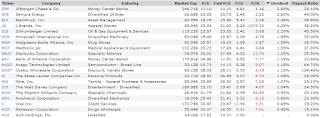## Pages

### 20 Best Stocks to Hold for the Long-Term

Forget about buying and selling stocks within a matter of days or months. Morgan Stanley is out with a new note recommending 30 companies that you should hold until 2018.

Below is a list of the 20 top dividend payers.

That's not to say you should then sell them in 2018, it's to say that Morgan Stanley believes these companies are poised to perform well over the next three years.

### JPMorgan Chase -- Yield: 2.63%

JPMorgan Chase (NYSE:JPM) employs 241,359 people, generates revenue of \$51,531.00 million and has a net income of \$21,762.00 million. The current market capitalization stands at \$248.68 billion.

JPMorgan Chase’s earnings before interest, taxes, depreciation and amortization (EBITDA) amounts to \$40,815.00 million. The EBITDA margin is 43.33% (the operating margin is 31.62% and the net profit margin 23.10%).

Financials: The total debt represents 21.98% of JPMorgan Chase assets and the total debt in relation to the equity amounts to 244.04%. Due to the financial situation, a return on equity of 9.76% was realized by JPMorgan Chase.

Twelve trailing months earnings per share reached a value of \$5.46. Last fiscal year, JPMorgan Chase paid \$1.58 in the form of dividends to shareholders.

Market Valuation: Here are the price ratios of the company: The P/E ratio is 12.18, the P/S ratio is 2.64 and the P/B ratio is finally 1.18. The dividend yield amounts to 2.63%.

Stock Charts:Long-Term Stock Price Chart of JPMorgan Chase (JPM)Long-Term Dividend Payment History of JPMorgan Chase (JPM)Long-Term Dividend Yield History of JPMorgan Chase (JPM)

### Sempra Energy -- Yield: 2.60%

Sempra Energy (NYSE:SRE) employs 17,046 people, generates revenue of \$11,018.00 million and has a net income of \$1,224.00 million. The current market capitalization stands at \$26.69 billion.

Sempra Energy’s earnings before interest, taxes, depreciation and amortization (EBITDA) amounts to \$2,907.00 million. The EBITDA margin is 26.38% (the operating margin is 16.40% and the net profit margin 11.11%).

Financials: The total debt represents 36.16% of Sempra Energy assets and the total debt in relation to the equity amounts to 126.64%. Due to the financial situation, a return on equity of 10.40% was realized by Sempra Energy.

Twelve trailing months earnings per share reached a value of \$5.38. Last fiscal year, Sempra Energy paid \$2.64 in the form of dividends to shareholders.

Market Valuation: Here are the price ratios of the company: The P/E ratio is 20.02, the P/S ratio is 2.42 and the P/B ratio is finally 2.34. The dividend yield amounts to 2.60%.

Stock Charts:Long-Term Stock Price Chart of Sempra Energy (SRE)Long-Term Dividend Payment History of Sempra Energy (SRE)Long-Term Dividend Yield History of Sempra Energy (SRE)

### BlackRock -- Yield: 2.35%

BlackRock (NYSE:BLK) employs 12,200 people, generates revenue of \$11,081.00 million and has a net income of \$3,264.00 million. The current market capitalization stands at \$61.09 billion.

BlackRock’s earnings before interest, taxes, depreciation and amortization (EBITDA) amounts to \$4,727.00 million. The EBITDA margin is 42.66% (the operating margin is 40.38% and the net profit margin 29.46%).

Financials: The total debt represents 3.47% of BlackRock assets and the total debt in relation to the equity amounts to 30.43%. Due to the financial situation, a return on equity of 12.24% was realized by BlackRock.

Twelve trailing months earnings per share reached a value of \$19.71. Last fiscal year, BlackRock paid \$7.72 in the form of dividends to shareholders.

Market Valuation: Here are the price ratios of the company: The P/E ratio is 18.79, the P/S ratio is 5.51 and the P/B ratio is finally 2.23. The dividend yield amounts to 2.35%.

Stock Charts:Long-Term Stock Price Chart of BlackRock (BLK)Long-Term Dividend Payment History of BlackRock (BLK)Long-Term Dividend Yield History of BlackRock (BLK)

### L Brands -- Yield: 2.27%

L Brands (NYSE:LB) employs 20,200 people, generates revenue of \$11,454.00 million and has a net income of \$1,042.00 million. The current market capitalization stands at \$25.77 billion.

L Brands’s earnings before interest, taxes, depreciation and amortization (EBITDA) amounts to \$2,391.00 million. The EBITDA margin is 20.87% (the operating margin is 17.05% and the net profit margin 9.10%).

Financials: The total debt represents 63.16% of L Brands assets and the total debt in relation to the equity amounts to 26,472.22%. Due to the financial situation, a return on equity of % was realized by L Brands.

Twelve trailing months earnings per share reached a value of \$3.50. Last fiscal year, L Brands paid \$1.36 in the form of dividends to shareholders.

Market Valuation: Here are the price ratios of the company: The P/E ratio is 25.34, the P/S ratio is 2.25 and the P/B ratio is finally 1,429.50. The dividend yield amounts to 2.27%.

Stock Charts:Long-Term Stock Price Chart of L Brands (LB)Long-Term Dividend Payment History of L Brands (LB)Long-Term Dividend Yield History of L Brands (LB)

### Schlumberger -- Yield: 2.21%

Schlumberger (NYSE:SLB) employs 120,000 people, generates revenue of \$48,631.00 million and has a net income of \$5,711.00 million. The current market capitalization stands at \$114.99 billion.

Schlumberger’s earnings before interest, taxes, depreciation and amortization (EBITDA) amounts to \$12,990.00 million. The EBITDA margin is 26.71% (the operating margin is 15.78% and the net profit margin 11.74%).

Financials: The total debt represents 19.92% of Schlumberger assets and the total debt in relation to the equity amounts to 35.22%. Due to the financial situation, a return on equity of 14.60% was realized by Schlumberger.

Twelve trailing months earnings per share reached a value of \$3.85. Last fiscal year, Schlumberger paid \$1.60 in the form of dividends to shareholders.

Market Valuation: Here are the price ratios of the company: The P/E ratio is 23.57, the P/S ratio is 2.36 and the P/B ratio is finally 3.05. The dividend yield amounts to 2.21%.

Stock Charts:Long-Term Stock Price Chart of Schlumberger (SLB)Long-Term Dividend Payment History of Schlumberger (SLB)Long-Term Dividend Yield History of Schlumberger (SLB)20 Best Stocks to Hold for the Long-Term (Click to enlarge), Source: long-term-investments.blogspot.com